# Mechanics

## Measurement of Velocity

Videos:

c4science: Measuring velocity with a motion sensor

Apparatus and Method:

Measurement of Velocity

Simulations: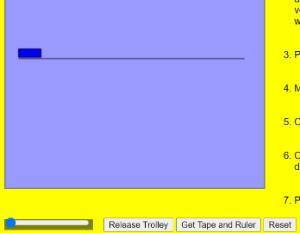Measurement of Velocity and AccelerationMotion with Constant Acceleration: This simulation is for motion with constant acceleration. For constant speed set acceleration to zero. Brooklyn College in New York have a good lab procedure for using this simulation to explore constant speed.

## Measurement of Acceleration

Videos:

Apparatus and Method:

Simulations:Measurement of Velocity and AccelerationMotion with Constant Acceleration: Brooklyn College in New York have a good lab procedure for using this simulation to explore constant velocity

## To show that a ∝ F

Videos:

Apparatus and Method:

Simulation: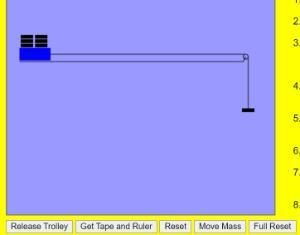To show that acceleration is proportional to force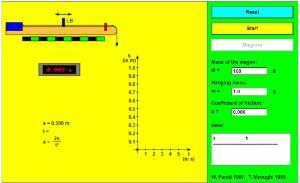Newton's Second Law Experiment: Brooklyn College in New York have a good lab procedure for using this simulation.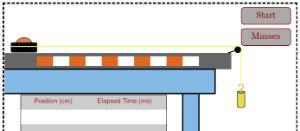Newton's Law Lab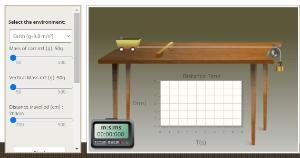Verification of Newton's Second Law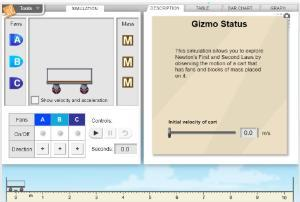Fan Cart Physics: Limited to 5 minutes

## Verification of the principle of conservation of momentum

Videos:

Apparatus and Method:

Verification of the principle of conservation of momentum

Simulations: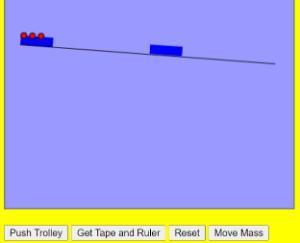Verification of the Principle of Conservation of Momentum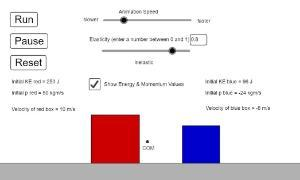Momentum & Energy: Elastic and Inelastic Collisions: Brooklyn College in New York have a good lab procedure for using this simulation.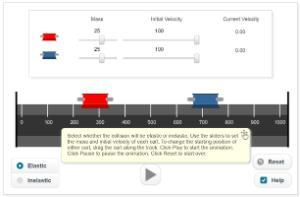Airtrack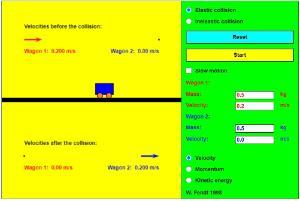Elastic and Inelastic Collision

## Measurement of g by freefall

Videos:

c4science: Measuring g with a free-fall apparatus

c4science: Measuring g with a picket fence

Simple Science and Maths: Measure Acceleration Due to Gravity

Noel Cunningham: Measuring acceleration due to gravity using a freefall apparatus

Apparatus and Method:

Verification of the principle of conservation of momentum

Simulations: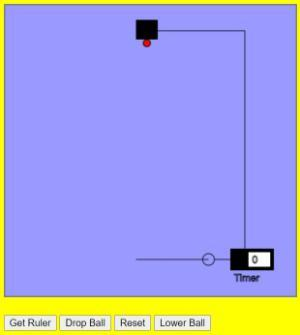To Measure Acceleration due to Gravity 'g' using a Free Fall apparatus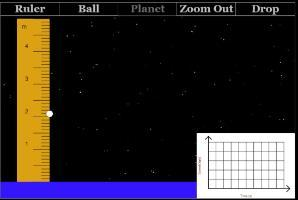Acceleration on a Planet Lab: Brooklyn College in New York have a good lab procedure (part 1) for using this simulation.Freefall: Brooklyn College in New York have a good lab procedure (part 2) for using this simulation.

## Verification of Boyle’s law

Videos:

c4science: Verifying Boyle's Law

PASCO: Boyle's Law

Noel Cunningham: Boyle's Law

Methods and Apparatus:

Verification of Boyle’s law

Simulations: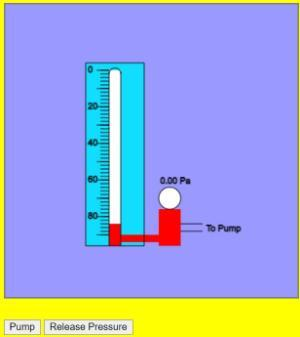Verification of Boyle's Law

## Investigation of the laws of equilibrium for a set of co-planar forces

Videos:

Apparatus and Method:

Investigation of the laws of equilibrium for a set of co-planar forces

Simulations:Investigation of the laws of equilibrium for a set of co-planar forces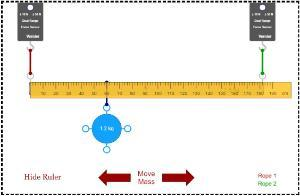Suspension Bridge Lab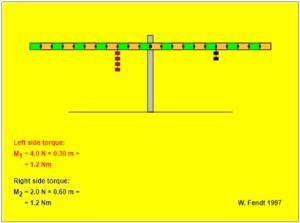Lever Principle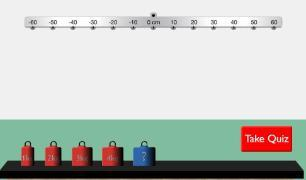Balance Beam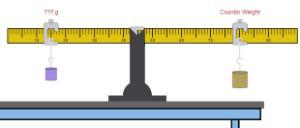Scale Lab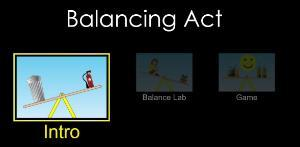Balancing Act

## Investigation of relationship between period and length for a simple pendulum and hence calculation of g

Videos:

Apparatus and Method:

Investigation of relationship between period and length for a simple pendulum

Simulations:Investigation of relationship between period and length of a Simple Pendulum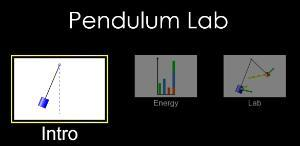Pendulum Lab: Brooklyn College in New York have a good lab procedure for using this simulation.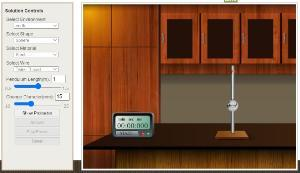Simple pendulum

## School of Physics

Scoil na Fisice

Room 213 (Physics Office), 2nd floor, Kane Science Building, University College Cork, Ireland.,

Top